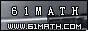Today is . WELCOME: huohai3983/44663  Home | Reg Login | All user | setHomePage | BookmarkHome | Math Test Online | Addition | Subtraction | Multiplication | Division | Time | Worksheet(JPG/PDF) | CAI | Flash Games |Recommendation articleArticle searchHome--maths--Third Grade--Mentally Adding Two-Digit Numbers

How to mentally add two two-digit numbers.

• First add the two ones' place digits (4 + 5 = 9).
• Next add the two tens' place digits (8 + 3 = 11).
• The sum of the ones' place digits is less than ten so the answer is 119
• First add the two ones' place digits (4 + 7 = 11).
• Next add the two tens' place digits (9 + 6 = 15).
• The sum of the ones' place digits is a two-digit number so
• decrease the ones' place sum by 10 (11 - 10 = 1)
• increase the tens' place sum by 1 (15 + 1 = 16)
• Combine the tens' and ones' place sums to give the answer of 161

Another method to mentally add two two-digit numbers may be easier in some situations.

• First add the two tens' place digits (8 + 3 = 11).
• Next add the two ones' place digits (4 + 5 = 9).
• The sum of the ones' place digits is less than ten so the answer is 119.
• First add the two tens' place digits (9 + 6 = 15).
• Next add the two ones' place digits (4 + 7 = 11).
• The sum of the ones' place digits is more than nine so:
• Decrease the ones' place sum by 10 (11 - 10 = 1.)
• Increase the tens' place sum by 1 (15 + 1 = 16).
• Combine the tens' and ones' place sums to give the answer of 161

#### Find the Sum of the two Numbers.

 +     =

2006-10-02 20:13:55
Viewed 3180 times CloseGreatmathsites